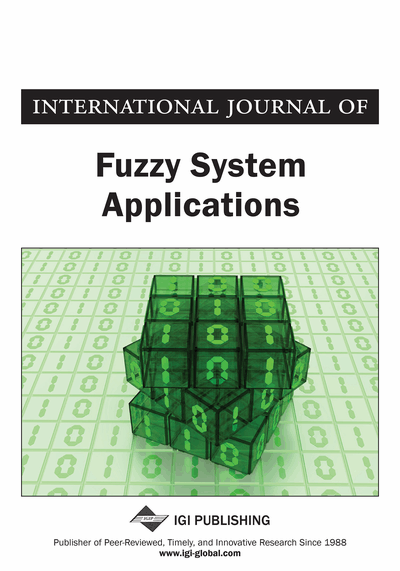# Dual Hesitant Fuzzy Soft Rings

V. Deepa
DOI: 10.4018/IJFSA.2018070101
OnDemand:
(Individual Articles)
Available
\$37.50
No Current Special Offers

## Abstract

In this article, the concept of dual hesitant fuzzy soft sets is applied to the subring and ideal structures of the classical rings. Further, based on level soft sets of the dual hesitant fuzzy soft set, a characterization theorem for the dual hesitant fuzzy soft ring is established. Moreover, a counter-example is provided for a dual hesitant fuzzy soft ring which is not an idealistic dual hesitant fuzzy soft ring. Finally, the homomorphic and bi-soft homomorphic properties of a dual hesitant fuzzy soft ring are discussed.
Article Preview
Top

## 1. Introduction

Molodtsov (Molodtsov, 1999) introduced soft set theory as a solution to the difficulty of lack of parameterization faced by the theory of fuzzy sets, the theory of interval mathematics and the theory of probability. The quality of parameterization makes soft set inevitable in the field of decision making. Torra (Torra, 2010) introduced Hesitant Fuzzy set to exhibit hesitation that arises when assigning membership degree to an element in a fuzzy set. As hesitation is a characteristic of human cognition, hesitant fuzzy set achieved a prominent role in decision-making field in a short span. To handle both epistemic certainty and epistemic uncertainty, Zhu et al. (Bin Zhu et al., 2012) proposed Dual hesitant fuzzy set. Further, Yan-ping He (Yan-ping He, 2016) combined Dual hesitant fuzzy set and Soft set forming a hybrid structure, Dual hesitant fuzzy soft set, thereby attaining the characteristics of both sets in a single framework. Later, Zhang et al. (Zhang et al., 2016) presented some operations on the dual hesitant fuzzy soft sets and discussed some of its properties.

During the paradigm shift which happened after the proposal of the Fuzzy set by Zadeh (Zadeh, 1965), exploring the algebraic structure of fuzzy sets and its extensions becomes an active and promising research area. In 1971, Rosenfield (Rosenfield, 1971) introduced the notion of fuzzy subgroups and subgroupoids. A book on fuzzy group theory written by Mordeson et al. (Mordeson et al., 2005) discussed theoretical aspects of fuzzy subgroups of a group in detail. Later Liu (Liu, 1982) introduced fuzzy ideals, fuzzy invariant subgroups, and fuzzy rings. After that Kuraoka (Kuraoka, 1992), Abou-Zaid (Abou-Zaid, 1993), Ren (Ren, 1985) and Liu et al. (Liu et al., 2001) studied about fuzzy quotient ring. In 2014, Deepak et al. (Deepak et al., 2014) studied about hesitant fuzzy subgroups and normal hesitant fuzzy subgroups. Moreover, the authors prove some homomorphism and isomorphism theorems in the hesitant fuzzy context.

Many researchers show an intense interest in applying soft sets to different algebraic structures. An attempt in this direction has been made by Y.B. Jun (Jun, 2008) by applying soft set to BCK/BCI algebra. In continuation of their work, Jun et al. (Jun et al., 2009) presented the notion of soft p-ideals and p-idealistic soft BCI-algebras. The introduction of an intersectional soft BCK/BCI algebras and ideals (Jun et al., 2012) are another important research in this direction. Later, in 2014, a similar study has been conducted by Jun et al. (Jun et al., 2014) in the framework of the hesitant fuzzy soft set.

In 2012, Aslam et al. (Aslam et al., 2012) extend the idea of soft groups introduced by Aktas and Cagman (Aktas et al., 2007). Besides, Acar et al. (Acar et al., 2010) defined the soft ring, soft ideal, and idealistic soft ring. Further, Liu et al. (Liu et al., 2008) studied the fuzzy soft group. Inan and Ozturk (Inan et al., 2012) extend this research to ring structures by introducing fuzzy soft ring and fuzzy soft ideals. Gosh et al. (Gosh et al., 2011) defined fuzzy soft rings and ideals based on t-norm and t-conorm. Zhimming Zhang (Zhimming Zhang, 2012) introduced intuitionistic fuzzy soft rings and idealistic intuitionistic fuzzy soft rings and further gave a characterization theorem for intuitionistic fuzzy soft rings.

## Complete Article List

Search this Journal:
Reset
Volume 13: 1 Issue (2024)
Volume 12: 1 Issue (2023)
Volume 11: 4 Issues (2022)
Volume 10: 4 Issues (2021)
Volume 9: 4 Issues (2020)
Volume 8: 4 Issues (2019)
Volume 7: 4 Issues (2018)
Volume 6: 4 Issues (2017)
Volume 5: 4 Issues (2016)
Volume 4: 4 Issues (2015)
Volume 3: 4 Issues (2013)
Volume 2: 4 Issues (2012)
Volume 1: 4 Issues (2011)
View Complete Journal Contents Listing